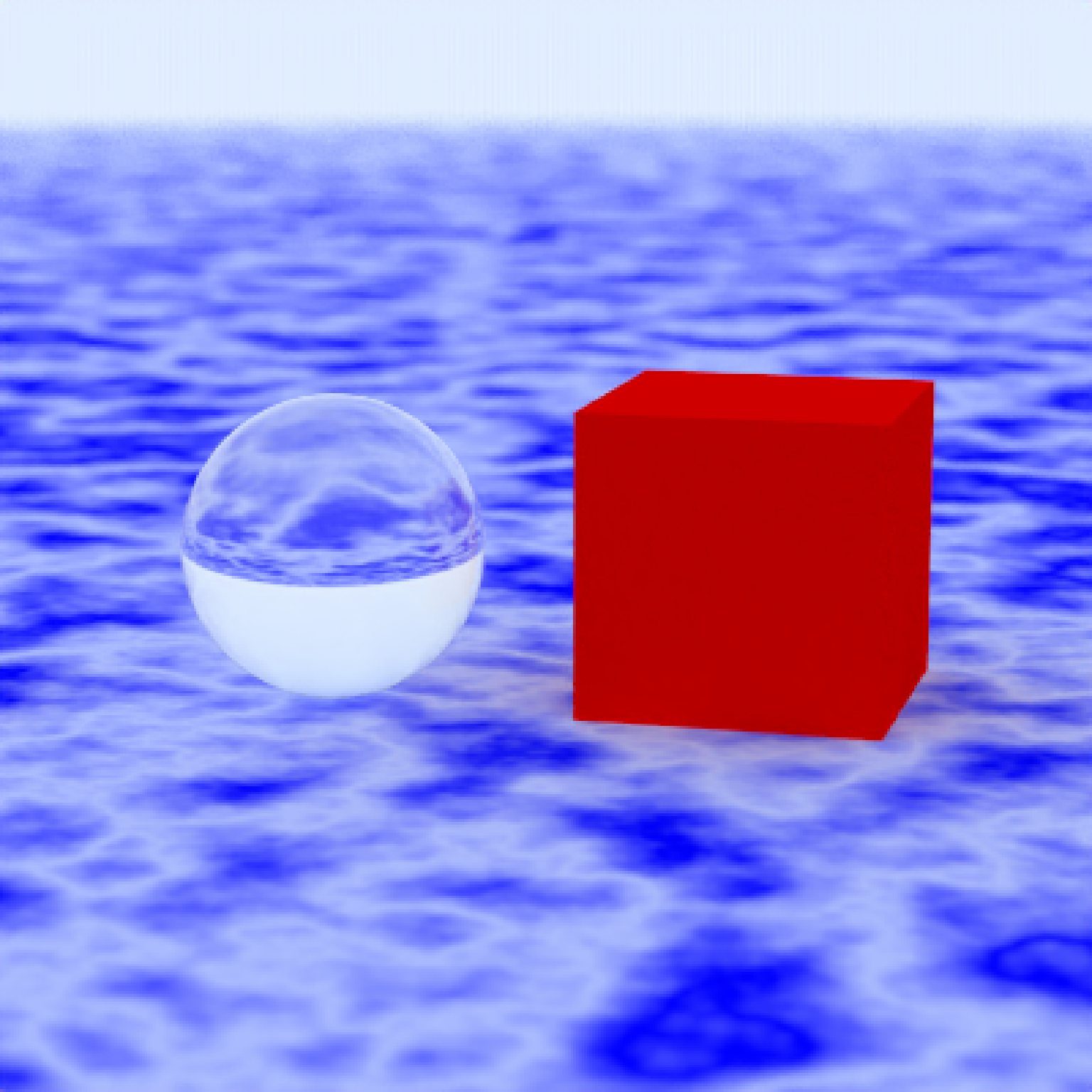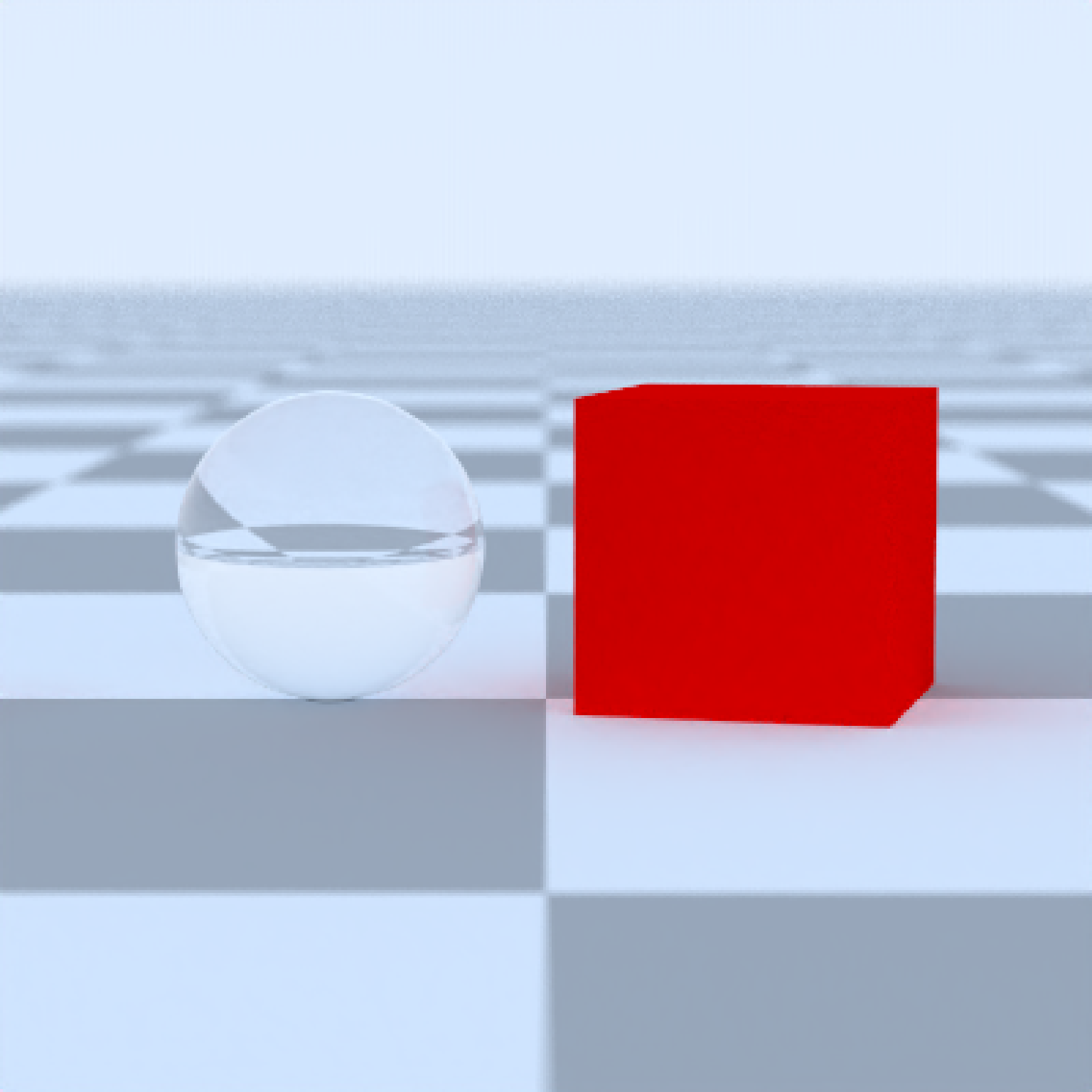Generates a large sphere that can be used as the ground for a scene.

generate_ground(
depth = -1,
spheresize = 1000,
material = diffuse(color = "#ccff00")
)

## Arguments

depth

Default -1. Depth of the surface.

spheresize

Default 1000. Radius of the sphere representing the surface.

material

Default diffuse with color= "#ccff00".The material, called from one of the material functions diffuse, metal, or dielectric.

color

Default #ccff00. The color of the sphere. Can be either a hexadecimal code, or a numeric rgb vector listing three intensities between 0 and 1.

## Value

Single row of a tibble describing the ground.

## Examples

#Generate the ground and add some objects
scene = generate_ground(depth=-0.5,
material = diffuse(noise=1,noisecolor="blue",noisephase=10)) %>%
if(run_documentation()) {
render_scene(scene, parallel=TRUE,lookfrom=c(0,2,10))
}# Make the sphere representing the ground larger and make it a checkered surface.
scene = generate_ground(depth=-0.5, spheresize=10000,
material = diffuse(checkercolor="grey50")) %>%